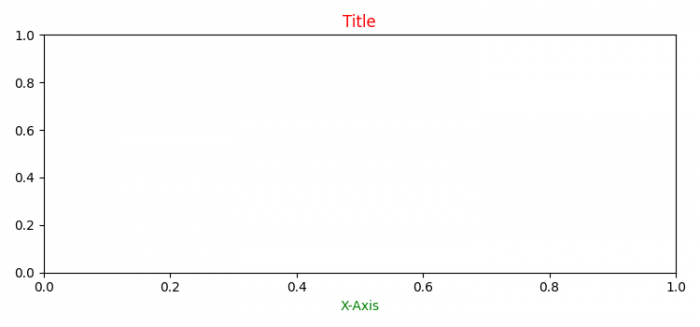# How do you change the default font color for all text in Matplotlib?

MatplotlibPythonData Visualization

#### Python Data Science basics with Numpy, Pandas and Matplotlib

Most Popular

63 Lectures 6 hours

#### Data Visualization using MatPlotLib & Seaborn

11 Lectures 4 hours

#### MatPlotLib with Python

9 Lectures 2.5 hours

To change the default font color for all text in Matplotlib, we can take the following steps −

• Set the figure size and adjust the padding between and around the subplots.
• Using rcParams['text.color'], we can get the default text color.
• We can update the text color and label color after updating the rcParams dict
• Set the title and label of the plot.
• To display the figure, use show() method.

## Example

import matplotlib.pyplot as plt

plt.rcParams["figure.figsize"] = [7.50, 3.50]
plt.rcParams["figure.autolayout"] = True

print("Default text color is: ", plt.rcParams['text.color'])
plt.rcParams.update({'text.color': "red",
'axes.labelcolor': "green"})
plt.title("Title")
plt.xlabel("X-axis")

plt.show()

## Output